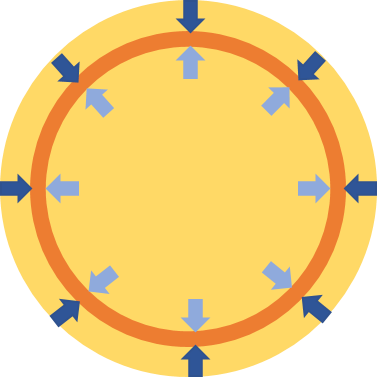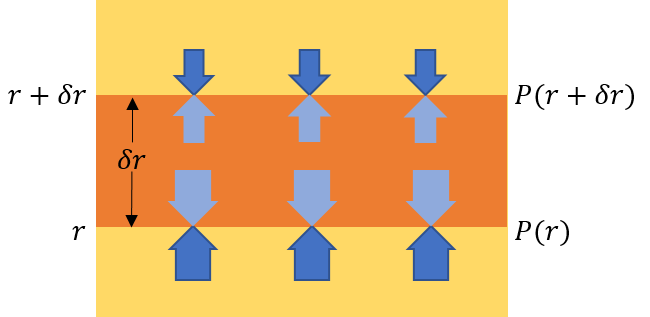# Stellar Equations: Hydrostatic Equilibrium

Friday, Feb 24, 2017
Section: Home / Post / Stellar equations
Categories: Physics,
Tags: Astronomy, Space, Astrophysics,

The star is stable. Despite being very massive, it does not collapse under its own gravity. This means that there is a force within the star that is opposing the inwards gravitational force. That force is a simple result of Newton’s third law: every action has an equal and opposite reaction.The inwards force of gravity is countered by the outwards reaction due to the pressure at that depth.

As gravity pushes inwards, the plasma inside the star is compressed. The deeper we go inside the star, the greater the pressure becomes since more and more of the star’s mass is pushing down on us. Just like the pressure increases as we dive deeper into water. And just like in water, the increasing pressure at greater depth generates a bouyancy force which counters our weight, making us float. This pressure gradient stabilizes the star against its own gravity.

Consider a parcel a distance $$r$$ from the center of the star. The parcel is of thickness $$\delta r$$. Let’s say the pressure is given by $$P(r)$$. We know that, by definition, the force experienced due to gravitational pressure at a distance $$r$$ from the center is:

$$\begin{equation}F(r) = P(r) \times A \end{equation}$$

Where $$A$$ is the area of the parcel bearing the weight of plasma pushing down. Since the pressure at the bottom of the parcel is greater, the bottom experiences more force compared to the top of the parcel. This creates a net upward force. This balances our the weight of the parcel - stablizing it.A parcel of plasma experiences forces on all sides due to pressure at that depth. Forces at the bottom are larger due to greater depth which generates a net upwards force.

Essentially:

$$\begin{equation}[P(r) - P(r+\delta r)] \times A = m\times g\end{equation}$$

Where $$g$$ is the strength of the gravitational field a distance $$r$$ from the center of the star, and $$m$$ is the mass of the parcel. However, we can simplify this equation further.

We can use Taylor’s Theorem to expand pressure at $$r+\delta r$$:

$$P(r+dr) \approx P(r) + \frac{dP}{dr} \times \delta r$$

On the right-hand-side of $$(2)$$ we can represent gravitational field strength as:

$$g = \frac{GM(r)}{r^{2}}$$

Including both of these simplifications gives us:

$$\begin{equation}[P(r) - (P(r) + \frac{dP}{dr} \times \delta r)] \times A = \frac{GM(r)}{r^{2}} \times m \end{equation}$$

$$-\frac{dP}{dr} \times \delta r \times A = \frac{GM(r)}{r^{2}} \times m$$

Where $$\delta r \times \delta A$$ is the volume of the parcel, and dividing my $$m$$ yields density $$\frac{1}{\rho (r)}$$:

$$-\frac{1}{\rho (r)}\frac{dP}{dr} = \frac{GM(r)}{r^{2}}$$

According to the Equation of Hydrostatic Equilibrium, the variation of pressure with depth in a star is proportional to the density and strength of gravity at that depth.